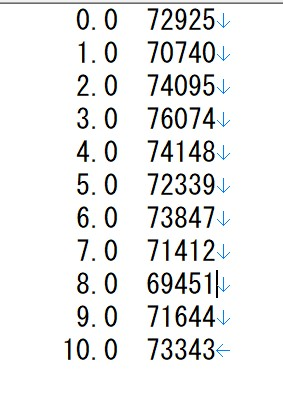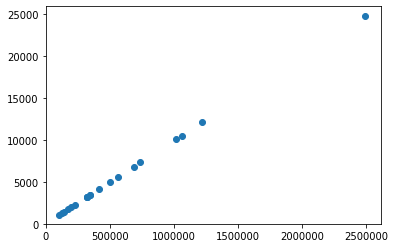# [PYTHON] How to read a serial number file in a loop, process it, and graph it

file_1.dat, file_2.dat ...... This is a method to read a dat file such as file_100.dat in which only numbers change continuously using a for statement, process it, and graph it. I couldn't find a cohesive explanation, so I'll leave it as a memorandum.

# What do you want to doThere are dozens of dat files with n rows and 2 columns like this, and I want to extract only the value in the second column, calculate it, and graph it. If you copy and paste one file at a time into Excel, it will take a lot of time and effort.

Initially, it was a program that read each file, processes it, assigns it to x, y values, and so on.

``````data_number_1,data_intensity_1 = np.loadtxt("file_1.dat",unpack = True)
s1 = su(data_intensity_1)
m1 = mean(data_intensity_1)
x=[s1]
y=[m1]
``````

It was a program that repeated this for the number of files, which was a ridiculously large number of lines and volumes, but as mentioned at the beginning, the file names differ only in the number part, so it is easy to use the for statement in Python. Can it be processed? That was the motivation for thinking about the program.

spyder(python3.7) Calculation: numpy (import numpy as np) Drawing: matplotrib (import matplotlib.pyplot as plt)

# Reading dat file and for statement

The sample code used for loading is as follows. Here, it is assumed that the serial number files from file_1 to file_20 are read.

``````data = []
for i in range(1,21):
``````

The code is explained step by step below.

## About the for statement

When setting the range of i in the for statement, the range of ** maximum value-1 ** is applied, so this time set the range as (1,21) as the file number 20 + 1.

Since the file name is file_ ** number **. Dat, use the format method and do it as ** file_ {} .dat ".format (i) **. By doing this, loop processing such as file_1.dat, file_2.dat ... can be performed.

Then, the value of the process repeated by ** data.append ** is added to the list called data that created these, and so on.

Of the dat file, I want to handle only the value on the second line as a numerical value, so I need to add processing for that.

If you read the numerical value using loadtext as it is, it will be put in the list line by line such as [0.0 72925], [1.0 70740] ... [10.0 73343] in the example of the previous image.

So I'll transpose it using ** unpack ** and put it in a different variable for each column. If you set it to True, transposition will occur, and the list can have different variables for each column, such as [0.0 1.0 ... 10.0], [72925 70740 ... 73343].

Also, since we want to treat only the second column as a numerical value without using the first column, specify the row to be read by ** usecols . Since we have decided which row to read 0 as the first row, we need to do it as ( 1 **) when reading the second column. That way, the data list will only contain [72925 70740 ... 73343].

For details, please see the reference site posted above. By using unpack and usecols in this way, I was able to put only the second column in a variable for each file.

data = [2nd column of file_1, 2nd column of file_2, ..., 2nd column of file_20].

In the original program, it was only that that was typed in the file branch! The number of lines and the amount of characters are surprisingly reduced. Of course, the more files you have, the easier it will be.

# processing

In this way, we were able to capture only the value on the second line in the entire file sentence, so next we will process each one. In order to process the values in the list at once, define a function and process them in order.

This time, let's assume that the horizontal axis is the sum of the values for each file, and the vertical axis is the average value.

Each function definition can be written as follows.

``````def su(a):
return np.sum(a)
``````
``````def mean(a):
return np.mean(a)
``````

Apply these processes individually to the list data.

``````for i in range(1,21):
si = su(data[i-1])
mi = mean(data[i-1])
``````

One thing to note here is the range value. Filenames start at 1, but list values start at 0. Therefore, when specifying the value of i in the function, it is necessary to do it as ** (i-1) **.

It means that you need to set the value that you think is 1 to 20 in the range of 0 to 19.

# Creating a graph

I will omit the detailed explanation this time. If you search with matplotrib, you will find various explanations.

It is necessary to set the values calculated by the above processing method as x and y, respectively. Therefore, make a list in the same way as when reading a file, and put the processed values in the x, y list in order.

``````x=[]
y=[]
for i in range(1,21):
si = sum(data[i-1])
mi = mean(data[i-1])
x.append(si)
y.append(mi)
``````

By doing this, we were able to prepare the values for the x-axis and y-axis, and the rest is completed by plotting using plot.

# Whole code sample

Based on the above, the whole code is as follows. (Values, files, and graphs are prepared as samples and have no meaning.)

``````import numpy as np
import matplotlib.pyplot as plt

def su(a):
return np.sum(a)

def mean(a):
return np.mean(a)

data = []
for i in range(1,21):

x=[]
y=[]
for i in range(1,21):
si = su(data[i-1])
mi = mean(data[i-1])
x.append(si)
y.append(mi)

plt.plot(x,y,ls="",marker="o");

plt.xlim(xmin=0)
plt.ylim(ymin=0)

plt.show()
``````By using loop processing in this way, we succeeded in simplifying more than 100 lines, and the efficiency has improved dramatically! I hope it will be helpful for those who are suffering from similar problems.

Thank you for reading.